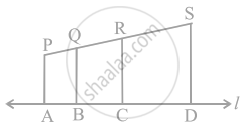# In figure, PA, QB, RC and SD are all perpendiculars to a line l, AB = 6 cm, BC = 9 cm, CD = 12 cm and SP = 36 cm. Find PQ, QR and RS. - Mathematics

Sum

In figure, PA, QB, RC and SD are all perpendiculars to a line l, AB = 6 cm, BC = 9 cm, CD = 12 cm and SP = 36 cm. Find PQ, QR and RS.#### Solution

Given, AB = 6 cm, BC = 9 cm, CD = 12 cm and SP = 36 cm

Also, PA, QB, RC and SD are all perpendiculars to line l

∴ PA || QB || RC || SD  .....[By Basic proportionality theorem]

PQ : QR : RS = AB : BC : CD = 6 : 9 : 12

Let PQ = 6x, QR = 9x and RS = 12x

Since, length of PS = 36 cm

∴ PQ + QR + RS = 36

⇒ 6x + 9x + 12x = 36

⇒ 27x = 36

∴ x = 36/27 = 4/3

Now, PQ = 6x = 6 xx 4/3 = 8 cm

QR = 9x = 9 xx 4/3 = 12 cm

And RS = 12x = 12 xx 4/3 = 16 cm

Concept: Basic Proportionality Theorem (Thales Theorem)
Is there an error in this question or solution?

#### APPEARS IN

NCERT Mathematics Exemplar Class 10
Chapter 6 Triangles
Exercise 6.4 | Q 14 | Page 75

Share